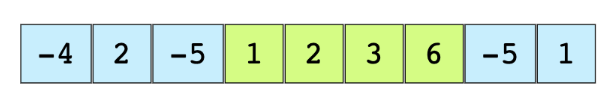# Largest Sum Subarray

## Problem Statement

In the array below, the largest sum subarray starts at index 3 and ends at 6, and with the largest sum being 12.## Try it yourself

int find_max_sum_sub_array(int A[], int n) {  //TODO: Write - Your - Code  return -1;}

## Solution

int find_max_sum_sub_array(int A[], int n) {  if (n < 1) {    return 0;  }    int curr_max = A;  int global_max = A;  for (int i = 1; i < n; ++i) {    if (curr_max < 0) {      curr_max = A[i];    } else {      curr_max += A[i];    }    if (global_max < curr_max) {      global_max = curr_max;    }  }  return global_max;}int main() {        int v[] = {-4, 2, -5, 1, 2, 3, 6, -5, 1};    cout << "Sum of largest subarray: " << find_max_sum_sub_array(v, sizeof(v) / sizeof(v)) << endl;    return 0;  }

## Solution Explanation

### Runtime complexity

The runtime complexity of this solution is linear, O(n).

### Memory complexity

The memory complexity of this solution is constant, O(1).

The basic idea of Kadane’s algorithm is to scan the entire array and at each position find the maximum sum of the subarray ending there. This is achieved by keeping a current_max for the current array index and a global_max. The algorithm is as follows:

current_max = A
global_max = A
for i = 1 -> size of A
if current_max is less than 0
then current_max = A[i]
otherwise
current_max = current_max + A[i]
if global_max is less than current_max
then global_max = current_max


Practice problems like this and many more by checking out our Grokking the Coding Interview: Patterns for Coding Questions course!

Learn in-demand tech skills in half the time